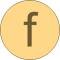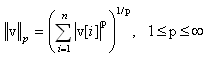# norm

Return the p-norm of a vector# Information

This information is part of the Modelica Standard Library maintained by the Modelica Association.

#### Syntax

```Vectors.norm(v);
Vectors.norm(v,p=2);   // 1 ≤ p ≤ ∞
```

#### Description

The function call "`Vectors.norm(v)`" returns the Euclidean norm "`sqrt(v*v)`" of vector v. With the optional second argument "p", any other p-norm can be computed:Besides the Euclidean norm (p=2), also the 1-norm and the infinity-norm are sometimes used:

 1-norm = sum(abs(v)) norm(v,1) 2-norm = sqrt(v*v) norm(v) or norm(v,2) infinity-norm = max(abs(v)) norm(v,Modelica.Constants.inf)

Note, for any vector norm the following inequality holds:

```norm(v1+v2,p) ≤ norm(v1,p) + norm(v2,p)
```

#### Example

```  v = {2, -4, -2, -1};
norm(v,1);    // = 9
norm(v,2);    // = 5
norm(v);      // = 5
norm(v,10.5); // = 4.00052597412635
norm(v,Modelica.Constants.inf);  // = 4
```